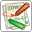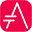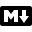# MathsWithin PlantUML, you can use AsciiMath notation:

@startuml :$int_0^1f(x)dx$; :$x^2+y_1+z_12^34$; note right Try also $d/dxf(x)=lim_(h->0)(f(x+h)-f(x))/h$ $P(y|bb"x") or f(bb"x")+epsilon$ end note @endumlor JLaTeXMath notation:

@startuml :<latex>\int_0^1f(x)dx</latex>; :<latex>x^2+y_1+z_{12}^{34}</latex>; note right Try also <latex>\dfrac{d}{dx}f(x)=\lim\limits_{h \to 0}\dfrac{f(x+h)-f(x)}{h}</latex> <latex>P(y|\mathbf{x}) \mbox{ or } f(\mathbf{x})+\epsilon</latex> end note @endumlHere is another example:

@startuml Bob -> Alice : Can you solve: $ax^2+bx+c=0$ Alice --> Bob: $x = (-b+-sqrt(b^2-4ac))/(2a)$ @enduml## Standalone diagramYou can also use @startmath/@endmath to create standalone AsciiMath formula.

@startmath f(t)=(a_0)/2 + sum_(n=1)^ooa_ncos((npit)/L)+sum_(n=1)^oo b_n\ sin((npit)/L) @endmathOr use @startlatex/@endlatex to create standalone JLaTeXMath formula.

@startlatex \sum_{i=0}^{n-1} (a_i + b_i^2) @endlatex## How is this working?To draw those formulas, PlantUML uses two open source projects:

• AsciiMath that converts AsciiMath notation to LaTeX expression;
• JLatexMath that displays mathematical formulas written in LaTeX. JLaTeXMath is the best Java library to display LaTeX code.

ASCIIMathTeXImg.js is small enough to be integrated into PlantUML standard distribution.

PlantUML relies on the Java Scripting API (specifically: new ScriptEngineManager().getEngineByName("JavaScript");) to load a JavaScript engine and execute JavaScript code. Java 8 includes a JavaScript engine called Nashorn but it was deprecated in Java 11.

If you are using AsciiMath in Java 11 you see the following warnings:

Warning: Nashorn engine is planned to be removed from a future JDK release

Nashorn was removed in Java 15. Fortunately, you can use the GraalVM JavaScript Engine instead by adding the following dependencies:

<dependency>
<groupId>org.graalvm.js</groupId>
<artifactId>js</artifactId>
<version>20.2.0</version>
</dependency>
<dependency>
<groupId>org.graalvm.js</groupId>
<artifactId>js-scriptengine</artifactId>
<version>20.2.0</version>
</dependency>


You can even use the GraalVM JavaScript Engine in Java 11 to get rid of the warning messages.

Since JLatexMath is bigger, you have to download it separately, then unzip the 4 jar files (batik-all-1.7.jar, jlatexmath-minimal-1.0.3.jar, jlm_cyrillic.jar and jlm_greek.jar) in the same folder as PlantUML.jar.